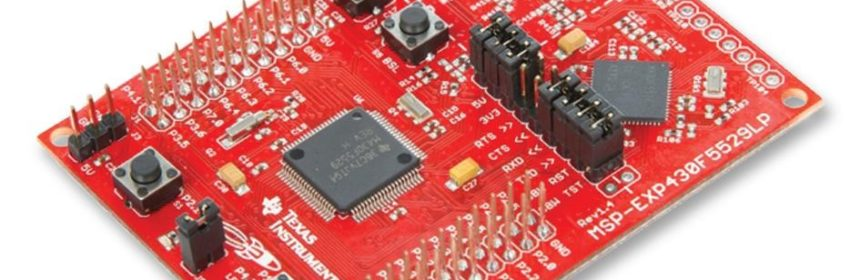## Tinkering TI MSP430F5529## Single Pulse Width Module (PWM) – TA2

PWM is an output feature of timers’ CC channels. Pulse width modulation (PWM) is needed in crafting switch-mode power supplies, inverters, motor controllers, servos and many other devices. PWM can also be used to generate analogue output similar to a Digital-to-Analogue Converter (DAC). In this example, we will see how we can use a single PWM channel to drive a servo motor.

### Code Example

```#include "driverlib.h"
#include "delay.h"

#define INC         1
#define DEC         0

void clock_init(void);
void GPIO_init(void);
void timer_T2A3_init(void);

void main(void)
{
unsigned char dir = INC;
unsigned int r = 0;

WDT_A_hold(WDT_A_BASE);

clock_init();
GPIO_init();
timer_T2A3_init();

while(true)
{
if((r  0) && (dir == DEC))
{
r--;
}

if((r == 0) && (dir == DEC))
{
dir = INC;
}

Timer_A_setCompareValue(TIMER_A2_BASE,
TIMER_A_CAPTURECOMPARE_REGISTER_1,
(1000 + r));

delay_ms(2);
};
}

void clock_init(void)
{
PMM_setVCore(PMM_CORE_LEVEL_3);

GPIO_setAsPeripheralModuleFunctionInputPin(GPIO_PORT_P5,
(GPIO_PIN4 | GPIO_PIN2));

GPIO_setAsPeripheralModuleFunctionOutputPin(GPIO_PORT_P5,
(GPIO_PIN5 | GPIO_PIN3));

UCS_setExternalClockSource(XT1_FREQ,
XT2_FREQ);

UCS_turnOnXT2(UCS_XT2_DRIVE_4MHZ_8MHZ);

UCS_turnOnLFXT1(UCS_XT1_DRIVE_0,
UCS_XCAP_3);

UCS_initClockSignal(UCS_MCLK,
UCS_XT2CLK_SELECT,
UCS_CLOCK_DIVIDER_1);

UCS_initClockSignal(UCS_SMCLK,
UCS_XT2CLK_SELECT,
UCS_CLOCK_DIVIDER_2);

UCS_initClockSignal(UCS_ACLK,
UCS_XT1CLK_SELECT,
UCS_CLOCK_DIVIDER_1);
}

void GPIO_init(void)
{
GPIO_setAsPeripheralModuleFunctionOutputPin(GPIO_PORT_P2,
GPIO_PIN4);
}

void timer_T2A3_init(void)
{
Timer_A_outputPWMParam outputPWMParam = {0};

outputPWMParam.clockSource = TIMER_A_CLOCKSOURCE_SMCLK;
outputPWMParam.clockSourceDivider = TIMER_A_CLOCKSOURCE_DIVIDER_2;
outputPWMParam.timerPeriod = 20000;
outputPWMParam.compareRegister = TIMER_A_CAPTURECOMPARE_REGISTER_1;
outputPWMParam.compareOutputMode = TIMER_A_OUTPUTMODE_RESET_SET;
outputPWMParam.dutyCycle = 0;

Timer_A_outputPWM(TIMER_A2_BASE,
&outputPWMParam);

Timer_A_setCompareValue(TIMER_A2_BASE,
TIMER_A_CAPTURECOMPARE_REGISTER_1,
1000);
}```

### Explanation

As mentioned, the goal of this demo is to drive a regular servo motor using a single PWM pin of MSP430F5529. Firstly, it must be noted that PWM or Capture-Compare function is a secondary function of GPIO pins. Thus, to use a PWM pin, it must be declared as Output Peripheral Module Function pin.

`GPIO_setAsPeripheralModuleFunctionOutputPin(GPIO_PORT_P2, GPIO_PIN4);`

Typical servos have a timing as shown below:

Note that every pulse has a period of 20ms. However, the high time varies from 5 – 10%, i.e. the duty cycle of the PWM should be between 5 – 10% in order to rotate the servo.

This time TA2 is used. We could have used Timer B as it is better suited for PWM. This won’t matter much though for this example.

```void timer_T2A3_init(void)
{
Timer_A_outputPWMParam outputPWMParam = {0};

outputPWMParam.clockSource = TIMER_A_CLOCKSOURCE_SMCLK;
outputPWMParam.clockSourceDivider = TIMER_A_CLOCKSOURCE_DIVIDER_2;
outputPWMParam.timerPeriod = 20000;
outputPWMParam.compareRegister = TIMER_A_CAPTURECOMPARE_REGISTER_1;
outputPWMParam.compareOutputMode = TIMER_A_OUTPUTMODE_RESET_SET;
outputPWMParam.dutyCycle = 0;

Timer_A_outputPWM(TIMER_A2_BASE, &outputPWMParam);

Timer_A_setCompareValue(TIMER_A2_BASE,TIMER_A_CAPTURECOMPARE_REGISTER_1, 1000);
}```

SMCLK is again used to clock the timer but this time it is scaled by a factor of 2. SMCLK will thus have a frequency of 2MHz as it is feed with 4MHz XT2_CLK.

`UCS_initClockSignal(UCS_SMCLK, UCS_XT2CLK_SELECT, UCS_CLOCK_DIVIDER_2);`

Inside the timer, this incoming clock signal is further divided by 2 and so the timer will tick at every 1µs. Like up mode, the top value or max PWM duty cycle is set to 20000. This means that the period of the PWM will be 20000 µs or 20 ms. Initially, the duty cycle is set to 0ms and the output mode is set to reset-set mode. Different output modes of MSP430’s PWM are shown below.

After setting the PWM parameters, the timer and its PWM are started. Initially the pulse high time is set to 1 ms, i.e. the servo will at 0° (-90°) position. We want the servo to rotate back and forth, i.e. from 0° (-90°) to 180° (+90°). To do this, we have to increase and decrease the pulse high time in the main loop.

```if((r  0) && (dir == DEC))
{
r--;
}
if((r == 0) && (dir == DEC))
{
dir = INC;
}
Timer_A_setCompareValue(TIMER_A2_BASE, TIMER_A_CAPTURECOMPARE_REGISTER_1, (1000 + r));

delay_ms(2);```

The line below is responsible of duty cycle variation:

`Timer_A_setCompareValue(TIMER_A2_BASE, TIMER_A_CAPTURECOMPARE_REGISTER_1, (1000 + r));`

It states which timer it is, which channel it is and the duty cycle of that channel.

### Related Posts

• Djalltralovely tutorial but to be honest I don’t think I’d be investing my time on this board to start with it’s not cheap and readily available as the stm32 boards can you please do more tutorials on stm32 board’s and the stc micros thanks

• GroouzzHello, I try to program MSP430FR6047 but i get error “the debug interface to the device has been secured”. when flashing using uniflash and when program using CCS this happen. can you help me to solve this problem

•You can try “On connect, erase user code and unlock the device” option.

• Pingback: Tinkering TI MSP430F5529 – gStore

• Surya k sHello
I am doing project of msp430g2553 interface(using i2c communication) with temp 100(temperature sensor) and try to read the temperature in dispaly(16*2) but didn’t get the out put (using code composer studio) can u share me any example code for this project

Thank you sir,

•Which sensor? Did you use pullup resistors for SDA-SCL pins?

• CristianWhere is lcd_print.h?

•You want the truth? TI makes and sell “underpowered micros”, you know? Low everything, not only the power but also peripherals. So the price is not justified.

Otherwise, if I’ll move there, I’ll introduce them to my small hobby projects – there are still some advantages.

•I may even make a visual configuration tool of my own for them…

•Yeah the prices of TI products are higher than other manufacturers but I don’t think the hardware peripherals are inferior.

•Not inferior but in not enough numbers compared to STM32.

•True